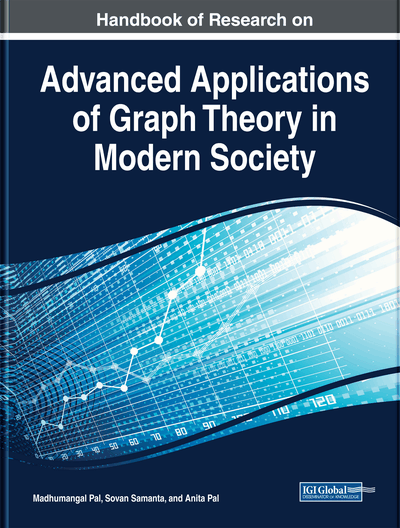# Energy of Graphs

Harishchandra S. Ramane (Karnatak University, India)
DOI: 10.4018/978-1-5225-9380-5.ch011
Available
\$37.50
No Current Special Offers

## Abstract

The energy of a graph G is defined as the sum of the absolute values of the eigenvalues of its adjacency matrix. The graph energy has close correlation with the total pi-electron energy of molecules calculated with Huckel molecular orbital method in chemistry. A graph whose energy is greater than the energy of complete graph of same order is called hyperenergetic graph. A non-complete graph having energy equal to the energy of complete graph is called borderenergetic graph. Two non-cospectral graphs are said to be equienergetic graphs if they have same energy. In this chapter, the results on graph energy are reported. Various bounds for graph energy and its characterization are summarized. Construction of hyperenergetic, borderenergetic, and equienergetic graphs are reported.
Chapter Preview
Top

## Introduction

The energy of a graph is the sum of the absolute values of the eigenvalues of its adjacency matrix. It has a correlation with the total π-electron energy of a molecule in the quantum chemistry as calculated with the Huckel molecular orbital method (Gutman & Polansky, 1986).

Let G be a finite, simple, undirected graph with vertex set V(G) and edge set F(G) The number of vertices of G is denoted by n and the number of edges of G is denoted by m. If V(G) = {v1, v2, ..., vn} then the adjacency matrix of G is a square matrix A(G) = [aij] of order n in which aij = 1, if the vertex vi is adjacent to the vertex vj and aij = 0, otherwise. The characteristic polynomial of A(G) denoted by Φ(G: λ) = det(λIA(G)), where I is an identity matrix of order n. The roots of the equation Φ(G: λ) = 0 are called the eigenvalues of G and they are labeled as λ1, λ2, ..., λn. Their collection is called the spectrum of G denoted by Spec(G) (Cvetkovic, Doob & Sachs, 1980).

If λ1, λ2, ..., λk are the distinct eigenvalues with respective multiplicities m1, m2, …, mk then we write Spec(G) =. Since A(G) is a real symmetric matrix, its eigenvalues are real and can be ordered as λ1 ≥ λ2 ≥ ... ≥ λn.

Two non-isomorphic graphs are said to be cospectral if they have same spectra. Details about the graph spectra can be found in the book (Cvetkovic, Doob & Sachs, 1980) and for graph theoretic terminology one can refer the book (Harary, 1999).

One of the chemical applications of spectral graph theory is based on the correspondence between the graph eigenvalues and the molecular orbital energy level of π-electron in conjugated hydrocarbons (Gutman & Polansky, 1986).

The molecular graph of a hydrocarbon is obtained as follows: the carbon atoms are represented by the vertices and two vertices are adjacent if and only if there is a carbon-carbon bond. Hydrogen atoms are ignored.

Within the Huckel molecular orbital (HMO) method (Huckel & Quantentheoretische Beitrage zum Benzolproblem, 1931), the energy level of π-electron in molecules of conjugated hydrocarbons are related to the eigenvalues of a molecular graph as εi = α + βi where α and β are empirical constants of the HMO model. The total energy of π-electrons denoted by Eπ is

,where gi is the occupation number with energy εi and g1 + g2 + … + gn = n. This yields

(1)

## Complete Chapter List

Search this Book:
Reset